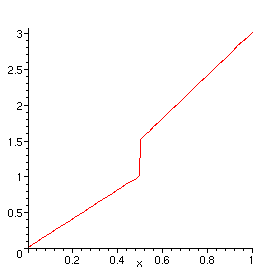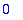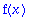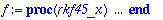Error: cannot evaluate boolean

Maple can't determine whether something is true or false in an "if" statement or "while" clause. This usually arises when a procedure that is meant to work for numeric arguments is called with symbolic arguments instead. It is often a case of "premature evaluation". Typical examples of this are

> f:= proc(x) if x > 1/2 then 3*x else 2*x fi end:

> plot(f(x), x = 0 .. 1);

Error, (in f) cannot evaluate boolean

> evalf(Int(f(x), x = 0 .. 1));

Error, (in f) cannot evaluate boolean

> fsolve(f(x) = 0, x);

Error, (in f) cannot evaluate boolean

The trouble is that Maple evaluates the inputs to a procedure such as plot before it calls the procedure, resulting in f being called with the symbolic argument x . The problem can be fixed as follows:

> plot('f(x)', x = 0 .. 1);> evalf(Int(f, 0 .. 1));> fsolve('f(x)' = 0, x);or modify the definition of f so that it accepts symbolic inputs (returning unevaluated), e.g.

> f:= proc(x)
if not type(x, numeric) then 'f(x)'
elif x > 0 then 3*x
else 2*x
fi
end:

> f(x);Another way this error can occur is in the numerical solution of a differential equation when the equation contains something symbolic (e.g. a parameter that has not been given a numerical value, or an undefined function). In some cases this arises by a misspelling. In the example below, the user probably intended to type sin rather than sim .

> f:= dsolve({D(y)(x)=sim(y(x)), y(0)=1},y(x),numeric);> f(3);

Error, (in dsolve/numeric/rkf45) cannot evaluate boolean